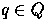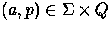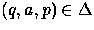Next: Remark Up: Basic Algorithms Previous: Remark

### Iteration on the outgoing transitions of a state

You will probably want to implement the following statement, for a:

for eachsuch thatdo something

Here is how to print on standard output the outgoing transition of a state:
#include "dfa_map.hh"
#include "tag.hh"
#include "alphabet.hh"
#include <iostream.h>

main()
{
typedef DFA_map<Range_alphabet<char, 'a', 'z'>, STag> DFA_type;
DFA_type dfa;
// Construct the automaton
// ....

// Prints the outgoing transitions of the initial state
DFA_type::State i = dfa.initial();
const DFA_type::Edges& edge = dfa.delta2(i);

DFA_type::Edges::const_iterator trans, trans_end = edge.end();
for (trans = edge.begin(); trans != trans_end; ++trans)
{
pair<DFA::Alphabet, DFA::State> t = *trans;
cout << t.first << " " << t.second << endl;
}
}


Vincent Lemaout
12/9/1997